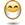Colebrook Equation

Excel Help for Colebrook Equation in Analyzing Manufacturing and Engineering Processes

Colebrook Equation

Rate this:
(3.5/5 from 2 votes)The Colebrook equation calculates the friction coefficient to determine pressure loss in ducts, tubes and pipes. The Colebrook friction coefficient is present on both sides of the equation requiring an iterative process to solve for it's value.

The equation is:
1/f1/2 = -2*Log(0.269541779*k/d+2.51*1/R/f1/2)/Log(10)

Where:
f = adjustment factor
k = roughness of surface
d = diameter
R = Reynolds number (ratio ratio of dynamic pressure and shearing stress)

We can calculate the Colebrook equation in Excel by setting up the following spreadsheet.

 A B C D 1 2 k 0.2 3 d 5.0 4 R 120,000 5 f 0.65 6 y =1/C5^0.5+2*LN(0.269541779*C2/C3+2.51*1/C4/C5^0.5)/LN(10) 7

The formula is in cell C5.  In order to solve the equation for the adjustment factor f in cell C5, we can employ the Solver Add-in.  The Solver Add-in can be activated by choosing Add-ins from the Excel menu (Tools > Add-ins for Excel prior to 2007 and Excel Options under the Office Button in Excel 2007 and higher).

To solve the equation the following parameters need to be set in the Solver dialogue:
• Set Target Cell: C6
• Equal To:, then  Value Of:, enter 0.0000001
• By Changing Cells: C5
• Subject To The Constraints: C5 < = 0.1 and C5 > = 0.0001
Click the Solve button to return the value for f that meets the equation constraints.